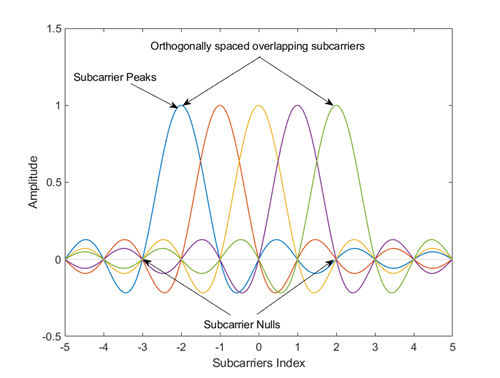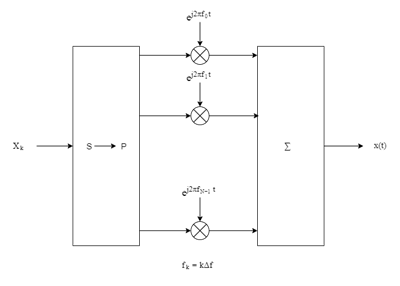# ofdmmod

Modulate frequency-domain signal using orthogonal frequency division multiplexing (OFDM)

## Syntax

``ofdmSig = ofdmmod(inSym,nfft,cplen)``
``ofdmSig = ofdmmod(inSym,nfft,cplen,nullidx)``
``ofdmSig = ofdmmod(inSym,nfft,cplen,nullidx,pilotidx,pilots)``

## Description

example

````ofdmSig = ofdmmod(inSym,nfft,cplen)` performs OFDM modulation on the frequency-domain input data subcarriers, `inSym`, using an FFT size specified by `nfft` and cyclic prefix length specified by `cplen`. For information, see OFDM Modulation.```

example

````ofdmSig = ofdmmod(inSym,nfft,cplen,nullidx)` inserts null subcarriers into the frequency domain input data signal prior to performing OFDM modulation. The null subcarriers are inserted at index locations from 1 to `nfft`, as specified by `nullidx`. For this syntax, the number of rows in the input `inSym` must be `nfft` – `length(nullidx)`. Use null carriers to account for guard bands and DC subcarriers. For information, see Subcarrier Allocation, Guard Bands and Guard Intervals.```

example

````ofdmSig = ofdmmod(inSym,nfft,cplen,nullidx,pilotidx,pilots)` inserts null and pilot subcarriers into the frequency domain input data symbols prior to performing OFDM modulation. The null subcarriers are inserted at the index locations specified by `nullidx`. The pilot subcarriers, `pilots`, are inserted at the index locations specified by `pilotidx`. For this syntax, the number of rows in the input `inSym` must be `nfft` – `length(nullidx)` – `length(pilotidx)`. The function assumes pilot subcarrier locations are the same across each OFDM symbol and transmit antenna.```

## Examples

collapse all

OFDM-modulate a fully packed input over two transmit antennas.

Initialize input parameters, generate random data, and perform OFDM modulation.

```nfft = 128; cplen = 16; nSym = 5; nt = 2; dataIn = complex(randn(nfft,nSym,nt),randn(nfft,nSym,nt)); y1 = ofdmmod(dataIn,nfft,cplen);```

Apply OFDM modulation assigning null subcarriers.

Initialize input parameters and generate random data.

```M = 16; % Modulation order for 16QAM nfft = 64; cplen = 16; nSym = 10; nullIdx = [1:6 33 64-4:64]'; numDataCarrs = nfft-length(nullIdx); inSig = randi([0 M-1],numDataCarrs,nSym);```

QAM modulate data. Perform OFDM modulation.

```qamSym = qammod(inSig,M,'UnitAveragePower',true); outSig = ofdmmod(qamSym,nfft,cplen,nullIdx);```

Perform OFDM modulation to input frequency domain data signal varying cyclic prefix length applied to each symbol.

Initialize input parameters and generate random data.

```M = 16; % Modulation order for 16QAM nfft = 64; cplen = [4 8 10 7 2 2 4 11 16 3]; nSym = 10; nullIdx = [1:6 33 64-4:64]'; numDataCarrs = nfft-length(nullIdx); inSig = randi([0 M-1],numDataCarrs,nSym);```

QAM modulate data. Perform OFDM modulation.

```qamSym = qammod(inSig,M,'UnitAveragePower',true); outSig = ofdmmod(qamSym,nfft,cplen,nullIdx);```

Apply OFDM modulation to a QPSK signal that is spatially multiplexed over two transmit antennas.

Initialize input parameters and generate random data for each antenna.

```M = 4; % Modulation order for QPSK nfft = 64; cplen = 16; nSym = 5; nt = 2; nullIdx = [1:6 33 64-4:64]'; pilotIdx = [12 26 40 54]'; numDataCarrs = nfft-length(nullIdx)-length(pilotIdx); pilots = repmat(pskmod((0:M-1).',M),1,nSym,2); ant1 = randi([0 M-1],numDataCarrs,nSym); ant2 = randi([0 M-1],numDataCarrs,nSym);```

QPSK modulate data individually for each antenna. Perform OFDM modulation.

```qpskSym(:,:,1) = pskmod(ant1,M); qpskSym(:,:,2) = pskmod(ant2,M); y1 = ofdmmod(qpskSym,nfft,cplen,nullIdx,pilotIdx,pilots);```

OFDM-modulate data input, specifying null and pilot packing.

Initialize input parameters, defining locations for null and pilot subcarriers. Generate random data and perform OFDM modulation.

```nfft = 64; cplen = 16; nSym = 10; nullIdx = [1:6 33 64-4:64]'; pilotIdx = [12 26 40 54]'; numDataCarrs = nfft-length(nullIdx)-length(pilotIdx); dataIn = complex(randn(numDataCarrs,nSym),randn(numDataCarrs,nSym)); pilots = repmat(pskmod((0:3).',4),1,nSym); y2 = ofdmmod(dataIn,nfft,cplen,nullIdx,pilotIdx,pilots);```

## Input Arguments

collapse all

Input data subcarriers, specified as an ND-by-NSym-by-NT array of symbols. The number of data subcarriers, ND, must equal `nfft``length(nullidx)``length(pilotidx)`. NSym is the number of OFDM symbols per transmit antenna, NT is the number of transmit antennas.

Input data symbols to an OFDM modulator are typically created with a baseband digital modulator, such as `qammod`.

Data Types: `double` | `single`
Complex Number Support: Yes

FFT length, specified as an integer greater than or equal to 8. `nfft` is equivalent to the number of subcarriers used in the modulation process.

Data Types: `double`

Cyclic prefix length, specified as a scalar or as a row vector of length NSym.

• When you specify `cplen` as a scalar, the cyclic prefix length is the same for all symbols through all antennas.

• When you specify `cplen` as a row vector of length NSym, the cyclic prefix length can vary across symbols but remains the same length through all antennas.

Data Types: `double`

Indices of null subcarrier locations, specified as a column vector with element values from 1 to `nfft`.

Data Types: `double`

Indices of pilot subcarrier locations, specified as a column vector with element values from 1 to `nfft`.

Data Types: `double`

Pilot subcarriers, specified as an NPilot-by-NSym-by-NT array of symbols. NPilot must equal the length of `pilotidx`. NSym is the number of OFDM symbols per transmit antenna. NT is the number of transmit antennas. The function assumes pilot subcarrier locations are the same across each OFDM symbol and transmit antenna. Use the `comm.OFDMModulator` to vary pilot subcarrier locations across OFDM symbols or antennas.

Data Types: `double` | `single`

## Output Arguments

collapse all

Modulated OFDM symbols, returned as a 2-D array of complex symbols.

• If `cplen` is a scalar, the array size is ((`nfft` + `cplen`) × NSym)-by-NT.

• If `cplen` is a row vector, the array size is ((`nfft` × NSym) + `sum`(`cplen`))-by-NT.

NSym is the number of symbols per transmit antenna and NT is the number of transmit antennas.

Data Types: `double` | `single`
Complex Number Support: Yes

collapse all

### OFDM Modulation

OFDM belongs to the class of multicarrier modulation schemes. Since the multiple data streams can be transmitted simultaneously with multiple carriers, OFDM is not influenced by noise to the same degree as single-carrier modulation.

OFDM operation divides a high-rate data stream into lower data rate substreams by decomposing the transmission frequency band into N contiguous individually modulated subcarriers. Multiple parallel and orthogonal subcarriers carry the samples with almost the same bandwidth as a wideband channel. By using narrow orthogonal subcarriers, the OFDM signal gains robustness over a frequency-selective fading channel and eliminates adjacent subcarrier interference. Intersymbol interference (ISI) is reduced because the lower data rate substreams have symbol durations larger than the channel delay spread.

The Frequency domain representation of orthogonal subcarriers in an OFDM waveform looks as follows:

``

The transmitter applies inverse fast Fourier transform (IFFT) to N symbols at a time. The output of the IFFT is the sum of the N orthogonal sinusoids:

`$x\left(t\right)=\sum _{k=0}^{N-1}{X}_{k}{e}^{j2\pi k\Delta ft},\text{ }0\le t\le T,$`

where {Xk} are data symbols, and T is the OFDM symbol time. The data symbols Xk are typically complex and can be from any digital modulation alphabet (for example, QPSK, 16-QAM, 64-QAM).

The subcarrier spacing is Δf = 1/T; ensuring that the subcarriers are orthogonal over each symbol period, as shown below:

`$\frac{1}{T}{\int }_{0}^{T}{\left({e}^{j2\pi m\Delta ft}\right)}^{*}\left({e}^{j2\pi n\Delta ft}\right)\text{\hspace{0.17em}}dt=\frac{1}{T}{\int }_{0}^{T}{e}^{j2\pi \left(m-n\right)\Delta ft}\text{\hspace{0.17em}}dt=0\text{ }\text{for}\text{\hspace{0.17em}}m\ne n.$`

An OFDM modulator consists of a serial-to-parallel conversion followed by a bank of N complex modulators, individually corresponding to each OFDM subcarrier.### Subcarrier Allocation, Guard Bands and Guard Intervals

Individual OFDM subcarriers are allocated as data, pilot, or null subcarriers.

As shown here, subcarriers are designated as data, DC, pilot, or guard band subcarriers.• Data subcarriers transmit user data.

• Pilot subcarriers are used for channel estimation.

• Null subcarriers transmit no data. Subcarriers with no data are used to provide a DC null and serve as buffers between OFDM resource blocks.

• The null DC subcarrier is the center of the frequency band with an index value of (`nfft`/2 + 1) if `nfft` is even, or ((`nfft` + 1) / 2) if `nfft` is odd.

• The guard bands provide buffers between consecutive OFDM symbols to protect the integrity of transmitted signals by reducing intersymbol interference.

Null subcarriers enable you to model guard bands and DC subcarrier locations for specific standards, such as the various 802.11 formats, LTE, WiMAX, or for custom allocations. You can allocate the location of nulls by assigning a vector of null subcarrier indices.

Similar to guard bands, guard intervals are used in OFDM to protect the integrity of transmitted signals by reducing intersymbol interference.

Assignment of guard intervals is analogous to the assignment of guard bands. You can model guard intervals to provide temporal separation between OFDM symbols. The guard intervals help preserve intersymbol orthogonality after the signal passes through time-dispersive channels. Guard intervals are created by using cyclic prefixes. Cyclic prefix insertion copies the last part of an OFDM symbol as the first part of the OFDM symbol.As long as the span of the time dispersion does not exceed the duration of the cyclic prefix, the benefit of cyclic prefix insertion is maintained.

Inserting a cyclic prefix results in a fractional reduction of user data throughput because the cyclic prefix occupies bandwidth that could be used for data transmission.

## Extended Capabilities

### Objects

Introduced in R2018a